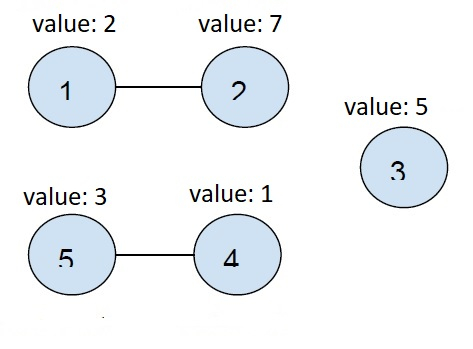# Sum of the minimum elements in all connected components of an undirected graph in C++

In this problem, we are given an array arr of N numbers where arr[i] represents (i+1)th node. Also, there are M pairs of edges where u and v represent the node connected by the edge. Our task is to create a program to find the sum of the minimum elements in all connected components of an undirected graph. If a node has no connectivity to any other node, count it as a component with one node.

Let’s take an example to understand the problem,

Input

arr[] = {2, 7, 5, 1, 2} m = 2
1 2
4 5

Output

8

Explanation

Below is the graph depicted above −We have two connected nodes and one single node

So, let’s take the minimum of all connected components

Min (node1 and node2) = min (2, 7) = 2

Min (node4 and node5) = min (1, 3) = 1

Min (node3) = min (5) = 5

Sum = 1 + 2 + 5 = 8

To solve this problem, we will find all connected nodes of the undirected graph using any of the traversal techniques (BFS or DFS) and then create a visited array for all nodes that are visited for that no double visit is not there. On visiting connected nodes that are connected directly or indirectly, we will find the minimum of all connections. And add this minimum value of thesum variable. After we have visited all the nodes, we will print the sum.

## Example

Program to illustrate the working of our solution,

Live Demo

#include <bits/stdc++.h>
using namespace std;
const int N = 100;
vector<int> graph[N];
bool visited[N];
void dfs(int node, int arr[], int minimum){
minimum = min(minimum, arr[node]);
visited[node] = true;
for (int i : graph[node]) {
if (!visited[i])
dfs(i, arr, minimum);
}
}
void createEdge(int u, int v){
graph[u - 1].push_back(v - 1);
graph[v - 1].push_back(u - 1);
}
int minSum(int arr[], int n){
int sum = 0;
for (int i = 0; i < n; i++) {
if (!visited[i]) {
int minimum = arr[i];
dfs(i, arr, minimum);
sum += minimum;
}
}
return sum;
}
int main(){
int arr[] = {2, 7, 5, 1, 3};
createEdge(1, 2);
createEdge(4, 5);
int n = sizeof(arr) / sizeof(arr);
cout<<"The sum of minimum elements in all connected components of an undirected graph is ";
cout<<minSum(arr, n);
return 0;
}

## Output

The sum of minimum elements in all connected components of an undirected graph is 8